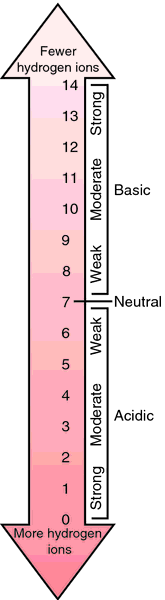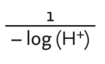# pH

(redirected from PH value)
Also found in: Dictionary, Thesaurus, Financial, Encyclopedia, Wikipedia.

## pH

the negative logarithm of the hydrogen ion concentration, [H+], a measure of the degree to which a solution is acidic or alkaline. An acid is a substance that can give up a hydrogen ion (H+); a base is a substance that can accept H+. The more acidic a solution the greater the hydrogen ion concentration and the lower the pH; a pH of 7.0 indicates neutrality, a pH of less than 7 indicates acidity, and a pH of more than 7 indicates alkalinity. The pH is used as a measure of whether the body is maintaining a normal acid-base balance. A favorable pH is essential to the functioning of enzymes and other biochemical systems. The body's fluids are normally somewhat alkaline, the pH being between 7.35 and 7.45. A pH above 7.8 or below 6.8 is generally fatal. See also acidosis and alkalosis.pH scale. From Applegate, 2000.

## Ph

Symbol for phenyl.

## pH

Symbol for the negative decadic logarithm of the H+ ion concentration (measured in moles per liter); a solution with pH 7.00 (1 × 10-7 g molecular weight of H+K per liter) is neutral at 22°C (that is, [H+] = [OH-]), one with a pH value of more than 7.00 is alkaline, one with a pH lower than 7.00 is acid. At 37°C, neutrality is at a pH value of 6.80. Compare: dissociation constant of water.
[p (power or potency) of [H+ ]]

## pH

the symbol relating the hydrogen ion (H+) concentration or activity of a solution to that of a given standard solution. Numerically the pH is approximately equal to the negative logarithm of H+ concentration expressed in molarity. pH 7 is neutral; above it alkalinity increases and below it acidity increases.

abbr.
1. penthouse
2. public health
3. Purple Heart

## pH

abbreviation for potential hydrogen, a scale representing the relative acidity (or alkalinity) of a solution, in which a value of 7.0 is neutral, below 7.0 is acid, and above 7.0 is alkaline. The numeric pH value indicates the relative concentration of hydrogen ions in the solution compared with that of a standard (1 molar) solution. It is equal to the negative log of the hydrogen ion concentration expressed in moles per liter. See also acid, acid-base balance.

## Ph

symbol or abbreviation for phenyl, C6H5.

## PH

Abbreviation for:
acidity (pH)
hydrogen ion concentration
papillary hyperplasia
parathyroid hormone
partial hysterectomy
passive haemagglutination
past history
patient history
peliosis hepatis
phaeochromocytoma
pharmacy
phenacetin
phosphate
pin hole
porphyria hepatica
posterior hypothalamus
postural hypotension
primary hyperparathyroidism
prolyl hydroxylase
Prospect Hill
pseudoepitheliomatous hyperplasia
public health
pulmonary haemorrhage
pulmonary haemosiderosis
pulmonary hypertension

## storage lesion

Transfusion medicine The constellation of changes occurring in a unit of packed red cells during storage. See Red cell preservatives.
Storage lesions
Ammonium to 470 µmol/L–US: 800 µg/dL
Free Hb in plasma from 82 to 6580 mg/L–US: 8.2 to 658 mg/dL
K+ from 4.2 to 78.5 mmol/L–US: 4.2 to 78.5 mEq/L
ATP from 100% to 45%
2,3 DPG to < 10% of original levels–replenished within 24 hours of transfusion
Labile proteins, eg complement, fibronectin and coagulation factors ↓ to negligible
Na+ from 169 to 111 mmol/L–US: 169 to 111 mEq/L
pH from 7.6 to 6.7
Adverse physiologic effects of stored blood is negligible in the absence of a previous compromise of the Pt's–recipient's status

## Ph

Abbreviation for phenyl.

## pH

Symbol for the negative logarithm of the H+ ion concentration (measured in moles per liter); a solution with pH 7.00 is neutral at 22°C, one with a pH of more than 7.0 is alkaline, and one with a pH lower than 7.00 is acid. At a temperature of 37°C, neutrality is at a pH value of 6.8.
[p (power or potency) of [H+]]

## Ph

1. Pharmacopoeia.
2. Symbol for phenyl.

## pH

[Ger. Potenz, power + H, symbol for hydrogen]
In chemistry, a measure of the hydrogen ion concentration of a solution. The degree of acidity or alkalinity of a substance is expressed in a pH value. A solution that is neither acid nor alkaline has a pH of 7. Increasing acidity is expressed as a number less than 7, and increasing alkalinity as a number greater than 7. Maximum acidity is pH 0 and maximum alkalinity is pH 14. Because the pH scale is logarithmic, there is a 10-fold difference between each unit. For example, pH 5 is 10 times as acid as pH 6 and pH 4 is 100 times as acid as pH 6. The general mathematical formula defining pH is: pH = -log [H+], in which pH is the negative logarithm of the hydrogen ion concentration. The pH of a solution may be determined electrically by a pH meter or colorimetrically by the use of indicators. A list of indicators and the pH range registered by each is given under the indicator.
See: illustration; table; indicatorHCl—hydrochloric acid; NaOH—sodium hydroxide
MaterialpH
10% HCl 1.0
Gastric juice 1.0–5.0
0.1% HCl 3.0
Pure water (neutral) at 25°C 7.0
Blood plasma 7.35–7.45
Pancreatic juice 8.4–8.9
0.1% NaOH11.0
10% NaOH13.0

## pH

An expression, widely used in medicine, of the acidity or alkalinity of a solution. pH is the logarithm to the base 10 of the concentration of free hydrogen ions in moles per litre, expressed as a positive number. The pH scale ranges from 0 to 14. Neutrality is 7. Figures below 7 indicate acidity, increasing towards zero; figures rising above 7 indicate increasing alkalinity. The pH of body fluids, in health, is accurately maintained between about 7.3 to 7.5. Below this range the condition of acidosis exists; above it, alkalosis. Both are dangerous.

## pH

a measure of the hydrogen ion concentration in an aqueous solution. The formula is:The formula produces a value in which the higher the number of H+ ions the lower the pH reading. The scale of pH values is from 1.0 (highly acid) to 14 (highly alkaline) with 7.0 as the neutral point. Since the pH is logarithmic, each change of one pH unit means a tenfold change in the number of hydrogen ions. pH can be measured by using indicators which change colour with changing pH, or by electrical means using a pH meter.

## pH

A measurement of the acidity or alkalinity of a solution based on the amount of hydrogen ions available. Based on a scale of 14, a pH of 7.0 is neutral. A pH below 7.0 is an acid; the lower the number, the stronger the acid. A pH above 7.0 is a base; the higher the number, the stronger the base. Blood pH is slightly alkaline (basic) with a normal range of 7.36-7.44.

## pH,

n measurement of the acidic or alkaline nature of a solution. Expressed as a numerical value, it is calculated by determining the log concentration of hydrogen ions present in the solution. A pH value of 7 indicates a neutral solution; a value less than 7 an acidic solution, and higher than 7 indicates an alkaline solution.

## pH

Symbol for the logarithm to base 10 of the reciprocal of the hydrogen (H) ion concentration measured in gram molecular weight in an aqueous solution. A solution with a pH 7.0 is neutral, one with a pH of more than 7.0 is alkaline, one with a pH lower than 7.0 is acid. It is a convenient way of expressing the acidity or alkalinity of solutions, particularly of contact lens buffer solutions. Alkaline ophthalmic solutions generally cause less discomfort than acidic ones. Note: pH stands for power (or potency) of H. See acidosis.

## pH

the negative logarithm of the hydrogen ion concentration [H+]; a measure of the degree to which a solution is acidic or alkaline. An acid is a substance that can give up a hydrogen ion (H+); a base is a substance that can accept H+. The more acidic a solution the greater the hydrogen ion concentration and the lower the pH; a pH of 7.0 indicates neutrality; a pH of less than 7 indicates acidity, and a pH of more than 7 indicates alkalinity.

pH-bicarbonate diagram
an aid to the assessment of an acid-base problem; expresses the relationship between bicarbonate ions and the pH of the plasma.
blood pH
normal blood pH varies a little between species but is of the order of 7.32 to 7.5. In moderate acidosis this falls to 7.25 to 7.30, severe acidosis 7.20 to 7.25 and grave acidosis to 7.00 to 7.10.
pH partition
the partition that occurs in the degree of ionization of electrolytes, including soluble drugs, about semipermeable membranes depending on the pH of the medium.
skin pH
in haired mammals, the pH of skin is usually acidic. In dogs it is from 5.5 to 7.2; in cats from 5.6 to 7.4; in cattle from 5.4 to 5.75; and in the horse from 4.8 to 6.8.
References in periodicals archive ?
The pH value of the conventional and nanofluids were measured using pH meter (Fig.
But, the reaction order depends on the initial pH values of phenol solution for both adsorbents.
It can be seen that the pH value decreases as the time increases.
Sorption efficiency in 4 mg/l formaldehyde solution (dependence on contact time and pH value with standard deviations)
2010a, b) found that the concentration of blood lactate was negatively correlated with the initial pH value (r = -0.
3M has received a patent for a composition comprised of an aqueous continuous liquid phase; and core-shell particles dispersed in the aqueous continuous liquid phase, each core-shell particle comprising a polymer core surrounded by a shell consisting essentially of nonporous spherical silica particles disposed on the polymer core, wherein the polymer core comprises at least one polymer, wherein a weight ratio of a total amount of the nonporous spherical silica particles in the composition to a total amount of the at least one polymer in the composition is in a range of from 85:15 to 95:5, wherein the nonporous spherical silica particles have a volume average particle diameter of 60 nanometers or less, and wherein the composition has a pH value of less than 5.
The experimental design involved 3 different pH levels (4, 5 and 7) and odonate nymphs were reared in each pH value.

Site: Follow: Share:
Open / Close